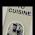## Sunday, 5 June 2011

### Age Calculation Formula

Hi,

I saw a question in developer community How to Calculate Age in formula field using Birth Date. It's reply was a very big formula

IF(MONTH(TODAY())> MONTH(DOB__c),YEAR(TODAY())-YEAR(DOB__c),IF(AND(MONTH(TODAY())= MONTH(DOB__c), DAY(TODAY())>= DAY(DOB__c)),YEAR(TODAY())-YEAR(DOB__c),(YEAR(TODAY())- YEAR(DOB__c))-1))

I Checked this this was working fine but It looked very complicated to me. So I thought of one formula that I used in first year of My Engg. in C Programming to determine age. In it we used to divide the date difference with 365.2425, why 365.2425 because we have leap years also so the avg. days in a year is not 365 but it is 365.2425 , So I created this new formula and it worked fine.

IF(ISNULL(DOB__c) , DOB__c , FLOOR((TODAY()-DOB__c +1)/365.2425))

I checked it, It is working fine in all cases , for leap year and all other cases that we usally check for age Calculation.

Regards

#### 1 comment:

1.Can you tell me the formulla of finding total experiance ?